# Stem and Leaf Plot

##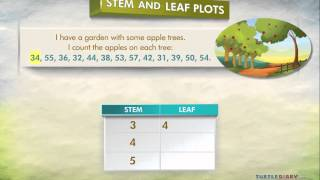By Turtlediary

A stem and leaf plot shows a large amount of data in a clear way by listing it in order of place value. A stem and leaf plot is generally used when data has multi-digit numbers. In this kind of plot, the ones digit of each number in the data is separated from the other digits. The plot has two columns. The first column, called the “stem”, lists all of the digits in the tens place and beyond, in order. A vertical line divides the stem from the “leaves” (the second column). This second column shows the ones place of each number in the data, also in order. Every number in the leaf column represents a number from the data. Its ones place is in the leaf, and the tens place and beyond are in the stem.# One-step equations with multiplication and division

##By Khan Academy

Let's get a conceptual understanding of why one needs to divide both sides of an equation to solve for a variable.# One-step equations with multiplication and division

##By Khan Academy

This equation can be simplified through a single step to solve for the variable. Can you help?# One-step equations with multiplication and division

##By Khan Academy

Let's ease into this, shall we? Here's an introduction to basic algebraic equations of the form ax=b. Remember that you can check to see if you have the right answer by substituting it for the variable!# One-step equations with multiplication and division

##By Khan Academy

Remember that what you do to one side, you have to do to the other. Will you multiply or divide both sides to dump the fraction, x/a? Let's do it together.# One-step equations with multiplication and division

##By Khan Academy

Let's ease into this, shall we? Here's an introduction to basic algebraic equations of the form ax=b. Remember that you can check to see if you have the right answer by substituting it for the variable!# One-step equations with multiplication and division

##By Khan Academy

Remember that what you do to one side, you have to do to the other. Will you multiply or divide both sides to dump the fraction, x/a? Let's do it together.# One-step equations with multiplication and division

##By Khan Academy

This equation can be simplified through a single step to solve for the variable. Can you help?# One-step equations with multiplication and division

##By Khan Academy

Let's get a conceptual understanding of why one needs to divide both sides of an equation to solve for a variable.# Estimating Quotients | MathHelp.com

##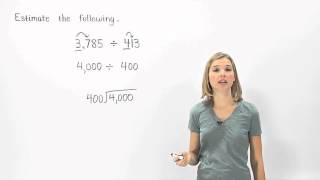By MathHelp.com

This lesson covers commutative property of multiplication, which states that a x b = b x a. For example, 10 x 4 = 4 x 10.# Dividing unit fractions by whole numbers

##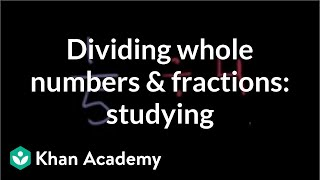By Khan Academy

Solve a word problem by dividing a fraction by a whole number. The example used in this video is 1/5 ï¿½ï¿½ï¿½ï¿½ï¿½ï¿½ï¿½ï¿½ï¿½ï¿½ï¿½ï¿½ 4.# Math Antics - Long Division

##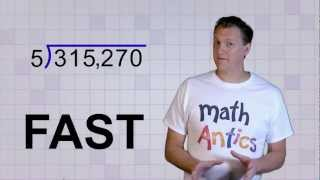By mathantics# Math Antics - Long Division with 2-Digit Divisors

##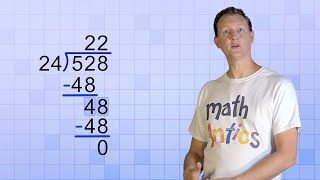By# Math Antics - Fractions and Decimals

##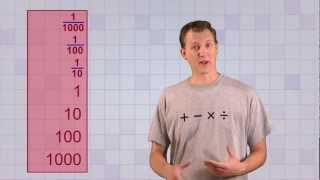By mathantics# Math Antics - Converting Any Fraction

##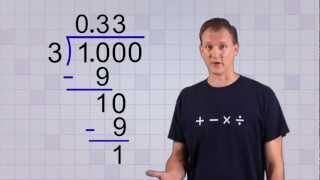By mathantics# Math Antics - Convert any Fraction to a Decimal

##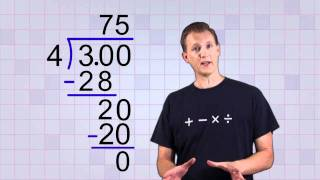By mathantics# Fast Math Trick - Long Division for Maths Reloaded

##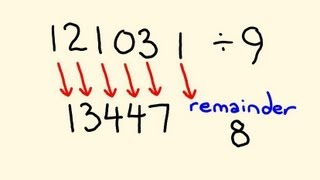By tecmath# Math Antics - Decimal Place Value

##By mathantics# Learn to Multiply Decimals (Decimal Multiplication) - 

##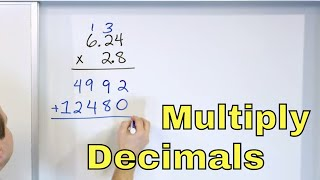By Math and Science# Math Antics - Decimal Arithmetic

##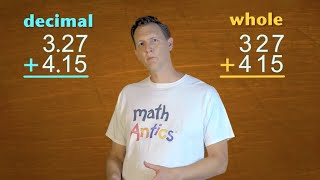By